# Stefan-Boltzmann Law

The thermal energy radiated by a blackbody radiator per second per unit area is proportional to the fourth power of the absolute temperature and is given byFor hot objects other than ideal radiators, the law is expressed in the form:where e is the emissivity of the object (e = 1 for ideal radiator). If the hot object is radiating energy to its cooler surroundings at temperature Tc, the net radiation loss rate takes the formThe Stefan-Boltzmann relationship is also related to the energy density in the radiation in a given volume of space.

### Development of the relationship

Index

Heat transfer concepts

 HyperPhysics***** Thermodynamics ***** Quantum Physics R Nave
Go Back

Thermal radiation is energy transfer by the emission of electromagnetic waves which carry energy away from the emitting object. For ordinary temperatures (less than red hot"), the radiation is in the infrared region of the electromagnetic spectrum. The relationship governing the net radiation from hot objects is called the Stefan-Boltzmann law: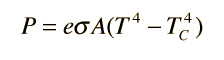CalculationWhile the typical situation envisioned here is the radiation from a hot object to its cooler surroundings, the Stefan-Boltzmann law is not limited to that case. If the surroundings are at a higher temperature (TC > T) then you will obtain a negative answer, implying net radiative transfer to the object.

 Why is a good absorber of radiation also a good emitter?
Index

Heat transfer concepts

 HyperPhysics***** Thermodynamics R Nave
Go Back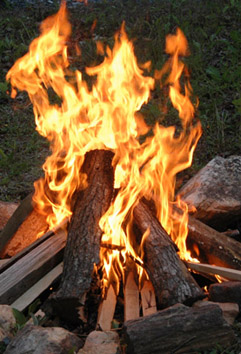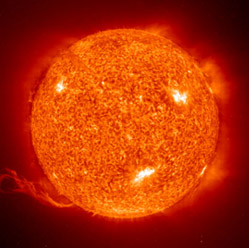The Sun at 5800K and a hot campfire at perhaps 800 K give off radiation at a rate proportional to the 4th power of the temperature.### Discussion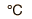=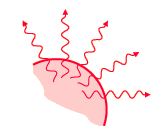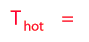=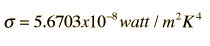Emissivity = (e = 1 for ideal radiator)= x 10^m2

=m2 = cm2 = ft2
 Radiation rate =x 10^watts

= watts
=calories/s = BTU/hr
After entering data, a calculation may be made by clicking on the highlighted symbol for the quantity you wish to calculate. A value for emissivity between 0 and 1 must be entered, with 1 representing a perfect radiator. The units for a given quantity will be reconciled when you enter data, but the relationship will not be forced to be consistent until you click on the quantity you desire to calculate.
 Modeling of cooling time
 Fraction of power in a wavelength range.
Index